# Time Constant Calculations

## DC Electric Circuits

• #### Question 1

 Don’t just sit there! Build something!!

Learning to mathematically analyze circuits requires much study and practice. Typically, students practice by working through lots of sample problems and checking their answers against those provided by the textbook or the instructor. While this is good, there is a much better way.

You will learn much more by actually building and analyzing real circuits, letting your test equipment provide the “answers” instead of a book or another person. For successful circuit-building exercises, follow these steps:

1. Carefully measure and record all component values prior to circuit construction.
2. Draw the schematic diagram for the circuit to be analyzed.
3. Carefully build this circuit on a breadboard or other convenient medium.
4. Check the accuracy of the circuit’s construction, following each wire to each connection point, and verifying these elements one-by-one on the diagram.
5. Mathematically analyze the circuit, solving for all values of voltage, current, etc.
6. Carefully measure those quantities, to verify the accuracy of your analysis.
7. If there are any substantial errors (greater than a few percent), carefully check your circuit’s construction against the diagram, then carefully re-calculate the values and re-measure.

Avoid very high and very low resistor values, to avoid measurement errors caused by meter “loading”. I recommend resistors between 1 kΩ and 100 kΩ, unless, of course, the purpose of the circuit is to illustrate the effects of meter loading!

One way you can save time and reduce the possibility of error is to begin with a very simple circuit and incrementally add components to increase its complexity after each analysis, rather than building a whole new circuit for each practice problem. Another time-saving technique is to re-use the same components in a variety of different circuit configurations. This way, you won’t have to measure any component’s value more than once.

• #### Question 2

The decay of a variable over time in an RC or LR circuit follows this mathematical expression:

 e−[t/(τ)]

Where,

e = Euler’s constant ( ≈ 2.718281828)

t = Time, in seconds

τ = Time constant of circuit, in seconds

For example, if we were to evaluate this expression and arrive at a value of 0.398, we would know the variable in question has decayed from 100% to 39.8% over the period of time specified.

However, calculating the amount of time it takes for a decaying variable to reach a specified percentage is more difficult. We would have to manipulate the equation to solve for t, which is part of an exponent.

Show how the following equation could be algebraically manipulated to solve for t, where x is the number between 0 and 1 (inclusive) representing the percentage of original value for the variable in question:

 x = e−[t/(τ)]

Note: the “trick” here is how to isolate the exponent [(−t)/(τ)]. You will have to use the natural logarithm function!

• #### Question 3

Re-write this mathematical expression so that the exponent term (−x) is no longer negative:

 e−x

Also, describe the calculator keystroke sequence you would have to go through to evaluate this expression given any particular value for x.

• #### Question 4

At a party, you happen to notice a mathematician taking notes while looking over the food table where several pizzas are set. Walking up to her, you ask what she is doing. Ï‘m mathematically modeling the consumption of pizza,” she tells you. Before you have the chance to ask another question, she sets her notepad down on the table and excuses herself to go use the bathroom.

Looking at the notepad, you see the following equation:

 Percentage = (e−[t/6.1]) ×100%

Where,

t = Time in minutes since arrival of pizza.

The problem is, you don’t know whether the equation she wrote describes the percentage of pizza eaten or the percentage of pizza remaining on the table. Explain how you would determine which percentage this equation describes. How, exactly, can you tell if this equation describes the amount of pizza already eaten or the amount of pizza that remains to be eaten?

• #### Question 5

The following two expressions are frequently used to calculate values of changing variables (voltage and current) in RC and LR timing circuits:

 e−[t/(τ)] or 1 − e−[t/(τ)]

One of these expressions describes the percentage that a changing value in an RC or LR circuit has gone from the starting time. The other expression describes how far that same variable has left to go before it reaches its ultimate value (at t = ∞).

The question is, which expression represents which quantity? This is often a point of confusion, because students have a tendency to try to correlate these expressions to the quantities by rote memorization. Does the expression e−[t/(τ)] represent the amount a variable has changed, or how far it has left to go until it stabilizes? What about the other expression 1 − e−[t/(τ)]? More importantly, how can we figure this out so we don’t have to rely on memory?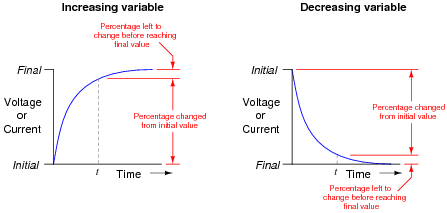• #### Question 6

Graph both the capacitor voltage (VC) and the capacitor current (IC) over time as the switch is closed in this circuit. Assume the capacitor begins in a complete uncharged state (0 volts):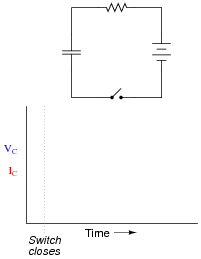Then, select and modify the appropriate form of equation (from below) to describe each plot:

 e−[t/(τ)] 1 − e−[t/(τ)]

• #### Question 7

The following circuit allows a capacitor to be rapidly charged and slowly discharged:Suppose that the switch was left in the “charge” position for some substantial amount of time. Then, someone moves the switch to the “discharge” position to let the capacitor discharge. Calculate the amount of capacitor voltage and capacitor current at exactly 3 seconds after moving the switch to the “discharge” position.

VC =

@ t = 3 seconds

IC =

@ t = 3 seconds

Also, show the direction of discharge current in this circuit.

• #### Question 8

The following circuit allows a capacitor to be rapidly discharged and slowly charged:Suppose that the switch was left in the “discharge” position for some substantial amount of time. Then, someone moves the switch to the “charge” position to let the capacitor charge. Calculate the amount of capacitor voltage and capacitor current at exactly 45 milliseconds after moving the switch to the “charge” position.

VC =

@ t = 45 ms

IC =

@ t = 45 ms

• #### Question 9

Qualitatively determine the voltages across all components as well as the current through all components in this simple RC circuit at three different times: (1) just before the switch closes, (2) at the instant the switch contacts touch, and (3) after the switch has been closed for a long time. Assume that the capacitor begins in a completely discharged state: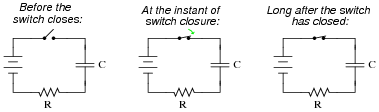Express your answers qualitatively: “maximum,” “minimum,” or perhaps “zero” if you know that to be the case.

Before the switch closes:

VC =

VR =

Vswitch =

I =

At the instant of switch closure:

VC =

VR =

Vswitch =

I =

Long after the switch has closed:

VC =

VR =

Vswitch =

I =

Hint: a graph may be a helpful tool for determining the answers!

• #### Question 10

Qualitatively determine the voltages across all components as well as the current through all components in this simple LR circuit at three different times: (1) just before the switch closes, (2) at the instant the switch contacts touch, and (3) after the switch has been closed for a long time.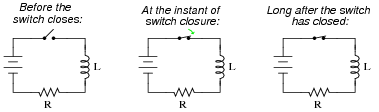Express your answers qualitatively: “maximum,” “minimum,” or perhaps “zero” if you know that to be the case.

Before the switch closes:

VL =

VR =

Vswitch =

I =

At the instant of switch closure:

VL =

VR =

Vswitch =

I =

Long after the switch has closed:

VL =

VR =

Vswitch =

I =

Hint: a graph may be a helpful tool for determining the answers!

• #### Question 11

Calculate the final value of current through the inductor with the switch in the left-hand position (assuming that many time constants’ worth of time have passed):Now, assume that the switch is instantly moved to the right-hand position. How much voltage will the inductor initially drop?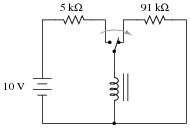Explain why this voltage is so very different from the supply voltage. What practical uses might a circuit such as this have?

• #### Question 12

An unfortunate tendency that many new students have is to immediately plug numbers into equations when faced with a time-constant circuit problem, before carefully considering the circuit. Explain why the following steps are very wise to follow before performing any mathematical calculations:

Step 1: Identify and list all the known (“given”) quantities.
Step 2: Draw a schematic of the circuit, if none is given to you.
Step 3: Label components in the schematic with all known quantities.
Step 4: Sketch a rough plot of how you expect the variable(s) in the circuit to change over time.
Step 5: Label starting and final values for these graphed variables, wherever possible.
• #### Question 13

Suppose a capacitor is charged to a voltage of exactly 100 volts, then connected to a resistor so it discharges slowly. Calculate the amount of voltage remaining across the capacitor terminals at the following points in time:

1 time constant (τ) after connecting the resistor:
2 time constants (2τ) after connecting the resistor:
3 time constants (3τ) after connecting the resistor:
4 time constants (4τ) after connecting the resistor:
5 time constants (5τ) after connecting the resistor:
• #### Question 14

Calculate the voltage across a 470 μF capacitor after discharging through a 10 kΩ resistor for 9 seconds, if the capacitor’s original voltage (at t = 0) was 24 volts.

Also, express this amount of time (9 seconds) in terms of how many time constants have elapsed.

• #### Question 15

Calculate the current through a 250 mH inductor after “charging” through a series-connected resistor with 100 Ω of resistance for 6 milliseconds, powered by a 12 volt battery. Assume that the inductor is perfect, with no internal resistance.

Also, express this amount of time (6 milliseconds) in terms of how many time constants have elapsed.

• #### Question 16

Determine the capacitor voltage at the specified times (time t = 0 milliseconds being the exact moment the switch contacts close). Assume the capacitor begins in a fully discharged state:Time VC (volts) 0 ms 30 ms 60 ms 90 ms 120 ms 150 ms

• #### Question 17

Plot the capacitor voltage and the capacitor current over time after the switch closes in this circuit, for at least 4 time constants’ worth of time:Be sure to label the axes of your graph!

• #### Question 18

Plot the inductor voltage and the inductor current over time after the switch closes in this circuit, for at least 4 time constants’ worth of time:Be sure to label the axes of your graph!

• #### Question 19

Calculate the amount of time it takes for a 33 μF capacitor to charge from 0 volts to 20 volts, if powered by a 24 volt battery through a 10 kΩ resistor.

• #### Question 20

Determine the amount of time needed after switch closure for the capacitor voltage (VC) to reach the specified levels: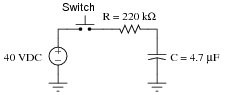VC Time 0 volts 10 volts 20 volts 30 volts 40 volts

Trace the direction of electron flow in the circuit, and also mark all voltage polarities.

• #### Question 21

A simple time-delay relay circuit may be built using a large capacitor connected in parallel with the relay coil, to temporarily supply the relay coil with power after the main power source is disconnected. In the following circuit, pressing the pushbutton switch sounds the horn, which remains on for a brief time after releasing the switch:To calculate the amount of time the horn will remain on after the pushbutton switch is released, we must know a few things about the relay itself. Since the relay coil acts as a resistive load to the capacitor, we must know the coil’s resistance in ohms. We must also know the voltage at which the relay “drops out” (i.e. the point at which there is too little voltage across the coil to maintain a strong enough magnetic field to hold the relay contacts closed).

Suppose the power supply voltage is 24 volts, the capacitor is 2200 μF, the relay coil resistance is 500 Ω, and the coil drop-out voltage is 6.5 volts. Calculate how long the time delay will last.

• #### Question 22

Design an experiment to calculate the size of a capacitor based on its response in a time-constant circuit. Include in your design an equation that gives the value of the capacitor in farads, based on data obtained by running the experiment.

• #### Question 23

A helpful technique for analyzing RC time-constant circuits is to consider what the initial and final values for circuit variables (voltage and current) are. Consider these four RC circuits:In each of these circuits, determine what the initial values will be for voltage across and current through both the capacitor and (labeled) resistor. These will be the voltage and current values at the very first instant the switch changes state from where it is shown in the schematic. Also, determine what the final values will be for the same variables (after a large enough time has passed that the variables are all “settled” into their ultimate values). The capacitor’s initial voltage is shown in all cases where it is arbitrary.

• #### Question 24

A helpful technique for analyzing LR time-constant circuits is to consider what the initial and final values for circuit variables (voltage and current) are. Consider these four LR circuits: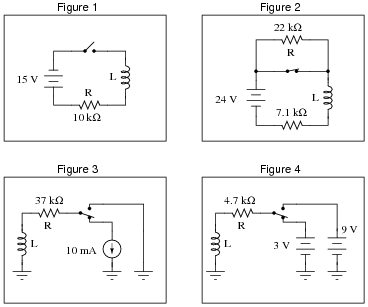In each of these circuits, determine what the initial values will be for voltage across and current through both the inductor and (labeled) resistor. These will be the voltage and current values at the very first instant the switch changes state from where it is shown in the schematic. Also, determine what the final values will be for the same variables (after a large enough time has passed that the variables are all “settled” into their ultimate values).

Assume all inductors are ideal, possessing no coil resistance (Rcoil = 0 Ω).

• #### Question 25

A formula I like to use in calculating voltage and current values in either RC or LR circuits has two forms, one for voltage and one for current:

 V(t) = ( Vf − V0 ) ( 1 − 1 e[t/(τ)] ) + V0 (for calculating voltage)

 I(t) = ( If − I0 ) ( 1 − 1 e[t/(τ)] ) + I0 (for calculating current)

The “0” subscript represents the condition at time = 0 (V0 or I0, respectively), representing the “initial” value of that variable. The “f” subscript represents the “final” or “ultimate” value that the voltage or current would achieve if allowed to progress indefinitely. Obviously, one must know how to determine the “initial” and “final” values in order to use either of these formulae, but once you do you will be able to calculate any voltage and any current at any time in either an RC or LR circuit.

What is not so obvious to students is how each formula works. Specifically, what does each portion of it represent, in practical terms? This is your task: to describe what each term of the equation means in your own words. I will list the “voltage” formula terms individually for you to define:

 V(t) =

 ( Vf − V0 ) =

 ( 1 − 1 e[t/(τ)] ) =

• #### Question 26

Determine the voltage across the capacitor three seconds after the switch is moved from the upper position to the lower position, assuming it had been left in the upper position for a long time:• #### Question 27

Calculate the voltage across the switch contacts the exact moment they open, and 15 milliseconds after they have been opened:• #### Question 28

The decay of a variable (either voltage or current) in a time-constant circuit (RC or LR) follows this mathematical expression:

 e−[t/(τ)]

Where,

e = Euler’s constant ( ≈ 2.718281828)

t = Time, in seconds

τ = Time constant of circuit, in seconds

Calculate the value of this expression as t increases, given a circuit time constant (τ) of 1 second. Express this value as a percentage:

t = 1 second
t = 2 seconds
t = 3 seconds
t = 4 seconds
t = 5 seconds
t = 6 seconds
t = 7 seconds
t = 8 seconds
t = 9 seconds
t = 10 seconds

Based on your calculations, how would you describe the change in the expression’s value over time as t increases?

• #### Question 29

The decay of a variable (either voltage or current) in a time-constant circuit (RC or LR) follows this mathematical expression:

 e−[t/(τ)]

Where,

e = Euler’s constant ( ≈ 2.718281828)

t = Time, in seconds

τ = Time constant of circuit, in seconds

Calculate the value of this expression as t increases, given a circuit time constant (τ) of 2 seconds. Express this value as a percentage:

t = 1 second
t = 2 seconds
t = 3 seconds
t = 4 seconds
t = 5 seconds
t = 6 seconds
t = 7 seconds
t = 8 seconds
t = 9 seconds
t = 10 seconds

Also, express the percentage value of any increasing variables (either voltage or current) in an RC or LR charging circuit, for the same conditions (same times, same time constant).

• #### Question 30

Write a mathematical expression for calculating the percentage value of any increasing variables (either voltage or current) in an RC or LR time-constant circuit.

Hint: the formula for calculating the percentage of any decreasing variables in an RC or LC time-constant circuit is as follows:

 e−[t/(τ)]

Where,

e = Euler’s constant ( ≈ 2.718281828)

t = Time, in seconds

τ = Time constant of circuit, in seconds

Here, the value of the expression starts at 1 (100%) at time = 0 and approaches 0 (0%) as time approaches ∞. What I’m asking you to derive is an equation that does just the opposite: start with a value of 0 when time = 0 and approach a value of 1 as time approaches ∞.

• #### Question 31

Calculating variables in reactive circuits using time-constant formulae can be time consuming, due to all the keystrokes necessary on a calculator. Even worse is when a calculator is not available! You should be prepared to estimate circuit values without the benefit of a calculator to do the math, though, because a calculator may not always be available when you need one.

Note that Euler’s constant (e) is approximately equal to 3. This is not a close approximation, but close enough for “rough” estimations. If we use a value of three instead of e’s true value of 2.718281828…, we may greatly simplify the “decay” time constant formula:

 Percentage of change ≈ 3−[t/(τ)]

Suppose that a capacitive discharge circuit begins with a full-charge voltage of 10 volts. Calculate the capacitor’s voltage at the following times as it discharges, assuming τ = 1 second:

t = 0 seconds ; EC =
t = 1 second ; EC =
t = 2 seconds ; EC =
t = 3 seconds ; EC =
t = 4 seconds ; EC =
t = 5 seconds ; EC =

Without using a calculator, you should at least be able to calculate voltage values as fractions if not decimals!

• #### Question 32

Calculating variables in reactive circuits using time-constant formulae can be time consuming, due to all the keystrokes necessary on a calculator. Even worse is when a calculator is not available! You should be prepared to estimate circuit values without the benefit of a calculator to do the math, though, because a calculator may not always be available when you need one.

Note that Euler’s constant (e) is approximately equal to 3. This is not a close approximation, but close enough for “rough” estimations. If we use a value of three instead of e’s true value of 2.718281828…, we may greatly simplify the “increasing” time constant formula:

 Percentage of change ≈ 1 − 3−[t/(τ)]

Suppose that a capacitive charging circuit begins fully discharged (0 volts), and charges to an ultimate value of 10 volts. Calculate the capacitor’s voltage at the following times as it discharges, assuming τ = 1 second:

t = 0 seconds ; EC =
t = 1 second ; EC =
t = 2 seconds ; EC =
t = 3 seconds ; EC =
t = 4 seconds ; EC =
t = 5 seconds ; EC =

Without using a calculator, you should at least be able to calculate voltage values as fractions if not decimals!

• #### Question 33

Determine the number of time constants (τ) that 7.5 seconds is equal to in each of the following reactive circuits:

RC circuit; R = 10 kΩ, C = 220 μF ; 7.5 sec =
RC circuit; R = 33 kΩ, C = 470 μF ; 7.5 sec =
RC circuit; R = 1.5 kΩ, C = 100 μF ; 7.5 sec =
RC circuit; R = 790 Ω, C = 9240 nF ; 7.5 sec =
RC circuit; R = 100 kΩ, C = 33 pF ; 7.5 sec =
LR circuit; R = 100 Ω, L = 50 mH ; 7.5 sec =
LR circuit; R = 45 Ω, L = 2.2 H ; 7.5 sec =
LR circuit; R = 1 kΩ, L = 725 mH ; 7.5 sec =
LR circuit; R = 4.7 kΩ, L = 325 mH ; 7.5 sec =
LR circuit; R = 6.2 Ω, L = 25 H ; 7.5 sec =
• #### Question 34

At a party, you happen to notice a mathematician taking notes while looking over the food table where several pizzas are set. Walking up to her, you ask what she is doing. Ï‘m mathematically modeling the consumption of pizza,” she tells you. Before you have the chance to ask another question, she sets her notepad down on the table and excuses herself to go use the bathroom.

Looking at the notepad, you see the following equation:

 Percentage = (1 − e−[t/5.8]) ×100%

Where,

t = Time in minutes since arrival of pizza.

The problem is, you don’t know whether the equation she wrote describes the percentage of pizza eaten or the percentage of pizza remaining on the table. Explain how you would determine which percentage this equation describes. How, exactly, can you tell if this equation describes the amount of pizza already eaten or the amount of pizza that remains to be eaten?

• #### Question 35

The following expression is frequently used to calculate values of changing variables (voltage and current) in RC and LR timing circuits:

 e−[t/(τ)] or 1 e[t/(τ)]

If we evaluate this expression for a time of t = 0, we find that it is equal to 1 (100%). If we evaluate this expression for increasingly larger values of time (t → ∞), we find that it approaches 0 (0%).

Based on this simple analysis, would you say that the expression e−[t/(τ)] describes the percentage that a variable has changed from its initial value in a timing circuit, or the percentage that it has left to change before it reaches its final value? To frame this question in graphical terms . . .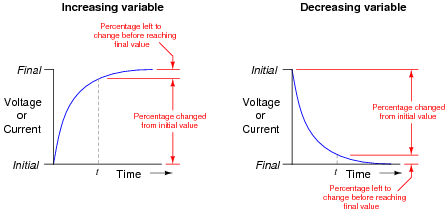Which percentage does the expression e−[t/(τ)] represent in each case? Explain your answer.

• #### Question 36

Determine the capacitor voltage and capacitor current at the specified times (time t = 0 milliseconds being the exact moment the switch contacts close). Assume the capacitor begins in a fully discharged state:Time VC (volts) IC (mA) 0 ms 30 ms 60 ms 90 ms 120 ms 150 ms

• #### Question 37

Determine the inductor voltage and inductor current at the specified times (time t = 0 milliseconds being the exact moment the switch contacts close):Time VL (volts) IL (mA) 0 μs 10 μs 20 μs 30 μs 40 μs 50 μs

• #### Question 38

Determine the inductor voltage and inductor current at the specified times (time t = 0 milliseconds being the exact moment the switch contacts close):Time VL (volts) IL (mA) 0 ns 250 ns 500 ns 750 ns 1.00 μs 1.25 μs

• #### Question 39

Complete this table of values for inductor voltage and current. Consider time = 0 to be the precise moment the switch closes:Time (μs) VL (V) IL (mA) 0 50 100 150 200 250 300 350 400

• #### Question 40

Calculate the voltage across a 2.5 H inductor after “charging” through a series-connected resistor with 50 Ω of resistance for 75 milliseconds, powered by a 6 volt battery. Assume that the inductor has an internal resistance of 14 Ω.

Also, express this amount of time (75 milliseconds) in terms of how many time constants have elapsed.

Hint: it would be helpful in your analysis to draw a schematic diagram of this circuit showing the inductor’s inductance and 14 ohms of resistance as two separate (idealized) components. This is a very common analysis technique in electrical engineering: to regard the parasitic characteristics of a component as a separate component in the same circuit.

• #### Question 41

Calculate the amount of time it takes for a 10 μF capacitor to discharge from 18 volts to 7 volts if its ultimate (final) voltage when fully discharged will be 0 volts, and it is discharging through a 22 kΩ resistor.

• #### Question 42

Determine the amount of time needed after switch closure for the capacitor voltage (VC) to reach the specified levels:VC Time 0 volts -5 volts -10 volts -15 volts -19 volts

Trace the direction of current in the circuit while the capacitor is charging, and be sure to denote whether you are using electron or conventional flow notation.

Note: the voltages are specified as negative quantities because they are negative with respect to (positive) ground in this particular circuit.

• #### Question 43

Determine the amount of time needed for the capacitor voltage (VC) to fall to the specified levels after the switch is thrown to the “discharge” position, assuming it had first been charged to full battery voltage:VC Time 10 volts 8 volts 6 volts 4 volts 2 volts

Trace the direction of electron flow in the circuit, and also mark all voltage polarities.

• #### Question 44

A submarine sonar system uses a “bank” of parallel-connected capacitors to store the electrical energy needed to send brief, powerful pulses of current to a transducer (a “speaker” of sorts). This generates powerful sound waves in the water, which are then used for echo-location. The capacitor bank relieves the electrical generators and power distribution wiring aboard the submarine from having to be rated for huge surge currents. The generator trickle-charges the capacitor bank, and then the capacitor bank quickly dumps its store of energy to the transducer when needed: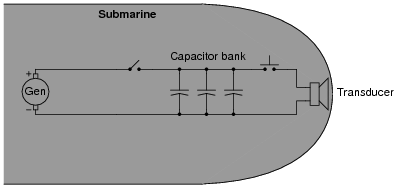As you might well imagine, such a capacitor bank can be lethal, as the voltages involved are quite high and the surge current capacity is enormous. Even when the DC generator is disconnected (using the “toggle” disconnect switch shown in the schematic), the capacitors may hold their lethal charge for many days.

To help decreases the safety risk for technical personnel working on this system, a “discharge” switch is connected in parallel with the capacitor bank to automatically provide a path for discharge current whenever the generator disconnect switch is opened:Suppose the capacitor bank consists of forty 1500 μF capacitors connected in parallel (I know the schematic only shows three, but . . .), and the discharge resistor is 10 kΩ in size. Calculate the amount of time it takes for the capacitor bank to discharge to 10 percent of its original voltage and the amount of time it takes to discharge to 1 percent of its original voltage once the disconnect switch opens and the discharge switch closes.

• #### Question 45

The model “555” integrated circuit is a very popular and useful “chip” used for timing purposes in electronic circuits. The basis for this circuit’s timing function is a resistor-capacitor (RC) network:In this configuration, the “555” chip acts as an oscillator: switching back and forth between “high” (full voltage) and “low” (no voltage) output states. The time duration of one of these states is set by the charging action of the capacitor, through both resistors (R1 and R2 in series). The other state’s time duration is set by the capacitor discharging through one resistor (R2):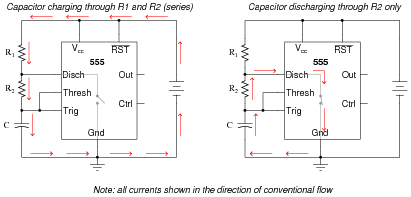Obviously, the charging time constant must be τcharge = (R1 R2)C, while the discharging time constant is τdischarge = R2C. In each of the states, the capacitor is either charging or discharging 50% of the way between its starting and final values (by virtue of how the 555 chip operates), so we know the expression e[(−t)/(τ)] = 0.5, or 50 percent.

Develop two equations for predicting the “charge” time and “discharge” time of this 555 timer circuit, so that anyone designing such a circuit for specific time delays will know what resistor and capacitor values to use.

### Footnotes:

For those who must know why, the 555 timer in this configuration is designed to keep the capacitor voltage cycling between 1/3 of the supply voltage and 2/3 of the supply voltage. So, when the capacitor is charging from 1/3VCC to its (final) value of full supply voltage (VCC), having this charge cycle interrupted at 2/3VCC by the 555 chip constitutes charging to the half-way point, since 2/3 of half-way between 1/3 and 1. When discharging, the capacitor starts at 2/3VCC and is interrupted at 1/3VCC, which again constitutes 50% of the way from where it started to where it was (ultimately) headed
.

• #### Question 46

Calculate the rate of change of voltage ([dv/dt]) for the capacitor at the exact instant in time where the switch moves to the “charge” position. Assume that prior to this motion the switch had been left in the “discharge” position for some time: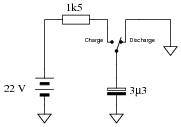• #### Question 47

Calculate the rate of change of current ([di/dt]) for the inductor at the exact instant in time where the switch moves to the “charge” position.• #### Question 48

Differential equations may be used to model the charging behavior of an RC circuit. Take, for instance, this simple RC circuit:We may develop a loop equation based on Kirchhoff’s Voltage Law, knowing that the voltage of the power source is constant (30 volts), and that the voltage drops across the capacitor and resistor are VC = Q/C and VR = IR, respectively:

 30 − IR − Q C = 0

To turn this into a true differential equation, we must express one of the variables as the derivative of the other. In this case, it makes sense to define I as the time-derivative of Q:

 30 − dQ dt R − Q C = 0

Show that the specific solution to this differential equation, assuming an initial condition of Q = 0 at t = 0, is as follows:

 Q = 0.0003(1 − e−50t)

Also, show this solution in a form where it solves for capacitor voltage (VC) instead of capacitor charge (Q).

• #### Question 49

Differential equations may be used to model the charging behavior of an L/R circuit. Take, for instance, this simple L/R circuit: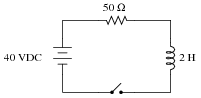We may develop a loop equation based on Kirchhoff’s Voltage Law, knowing that the voltage of the power source is constant (40 volts), and that the voltage drops across the inductor and resistor are VL = L[dI/dt] and VR = IR, respectively:

 40 − IR − L dI dt = 0

Show that the specific solution to this differential equation, assuming an initial condition of I = 0 at t = 0, is as follows:

 I = 0.8(1 − e−25t)

• #### Question 50

Assume that the switch in this circuit is toggled (switched positions) once every 5 seconds, beginning in the “up” (charge) position at time t = 0, and that the capacitor begins in a fully discharged state at that time. Determine the capacitor voltage at each switch toggle:Time Switch motion VC (volts) 0 s discharge → charge 0 volts 5 s charge → discharge 10 s discharge → charge 15 s charge → discharge 20 s discharge → charge 25 s charge → discharge

• #### Question 51

This passive integrator circuit is powered by a square-wave voltage source (oscillating between 0 volts and 5 volts at a frequency of 2 kHz). Determine the output voltage (vout) of the integrator at each instant in time where the square wave transitions (goes from 0 to 5 volts, or from 5 to 0 volts), assuming that the capacitor begins in a fully discharged state at the first transition (from 0 volts to 5 volts):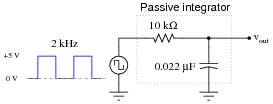Transition vout #1 (0 → 5 volts) 0 volts #2 (5 → 0 volts) #3 (0 → 5 volts) #4 (5 → 0 volts) #5 (0 → 5 volts) #6 (5 → 0 volts) #7 (0 → 5 volts) #8 (5 → 0 volts)

• #### Question 52

A 470 μF capacitor begins in a charged state of 270 volts, and discharges through a 100 kΩ resistor. How long will it take before the capacitor’s voltage will fall to a relatively safe value (30 volts or less)?

### Related Content

Published under the terms and conditions of the Creative Commons Attribution License•eetechzac November 15, 2021